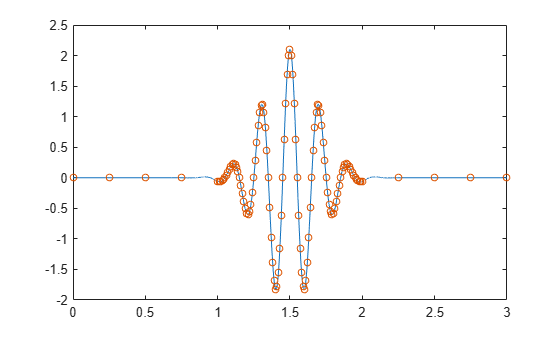# Create Uniform and Nonuniform Time Vectors

You can create uniform and nonuniform time vectors for use in computations involving time series.

### Uniform Time Vectors

Use the colon operator if you know the sampling frequency. If your system samples time at a rate of 15 Hz during one second, you get 16 readings, including the one at zero.

```Fs = 15; Ts = 1/Fs; ts = 0:Ts:1;```

Use `linspace` if you know the beginning and end of the time interval and the number of samples. Suppose you start a stopwatch and stop it one second later. If you know your instrument took 15 readings, you can generate the time vector.

`tl = linspace(0,1,15);`

You can compute the sample rate directly from the samples and use it to reconstruct the time vector.

```sf = 1/(tl(2)-tl(1)); TL = (0:length(tl)-1)/sf; ErrorTL = max(abs(tl-TL))```
```ErrorTL = 0 ```

You can also reconstruct `ts` using `linspace`.

```lts = length(ts); TS = linspace(ts(1),ts(lts),lts); ErrorTS = max(abs(ts-TS))```
```ErrorTS = 1.1102e-16 ```

`linspace` and the colon operator create row vectors by default. Transpose them to obtain column vectors.

```tcol = tl'; ttrans = ts';```

### Nonuniform Time Vectors

Combine `linspace` and the colon operator to generate nonuniform time vectors of arbitrary characteristics.

Suppose you have a Gaussian-modulated sinusoidal pulse that you must sample. The pulse changes rapidly during a one-second interval but slowly during the preceding and following seconds.

Sample the region of interest at 100 Hz and take only five samples before and after. Concatenate the vectors using square brackets.

```gpl = @(x) 2.1*gauspuls(x-1.5,5,0.4); Ffast = 100; Tf = 1/Ffast; Nslow = 5; tdisc = [linspace(0,1,Nslow) 1+Tf:Tf:2-Tf linspace(2,3,Nslow)];```

Generate 20001 samples of the function to simulate the continuous-time pulse. Overlay a plot of the samples defined by `tsf`.

```Tcont = linspace(0,3,20001)'; plot(Tcont,gpl(Tcont),tdisc,gpl(tdisc),'o','markersize',5)```